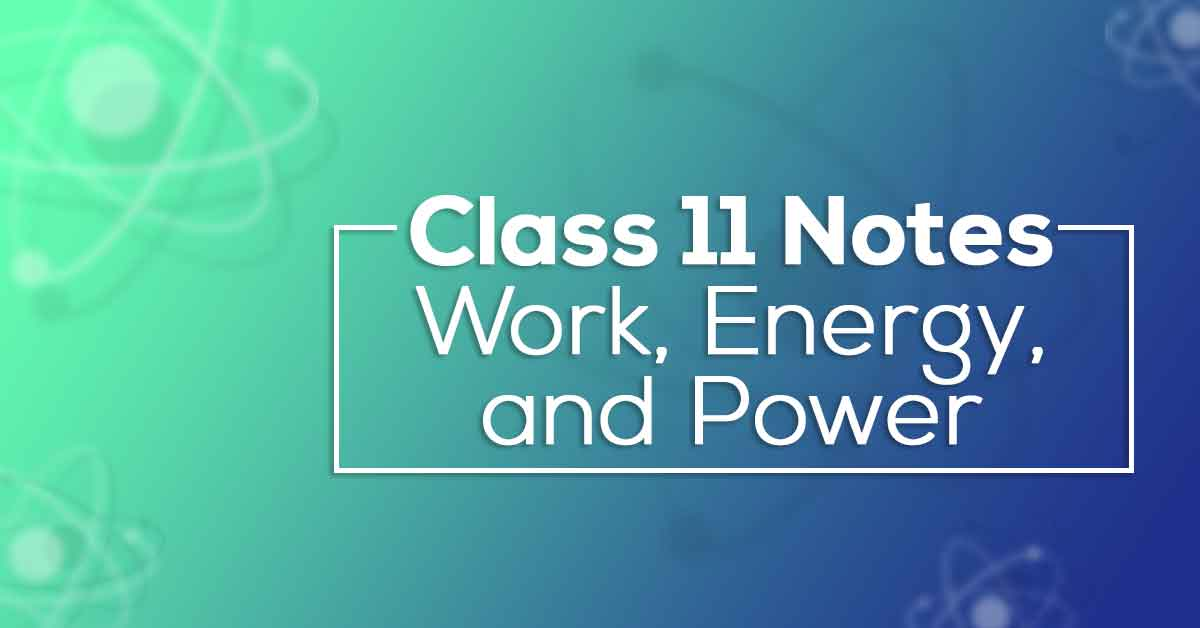Class 11 Physics Chapter 6 Work, Energy and Power Notes | Vidyakul
×

# Class 11 Physics Chapter 6 Work, Energy and Power Notes## Class 11 Physics Chapter 6 Work, Energy and Power Notes - PDF Download

Class 11 Physics Chapter 6 Work, Energy and Power Notes are prepared by our panel of highly qualified teachers who follow strict CBSE guidelines to get rid of any confusion among children regarding the content of the course since CBSE keeps on updating the course every year. These notes are made out of the past 10 years question papers and study material which help the students cover each and every concept given in the chapter. Work, Energy and Power Class 11 Notes would provide you with clear understanding and retention of the course content which would help you score more marks.

### What do you understand by Work, Energy and Power?

The work done by the force is defined to be the product of component of the force in the direction of the displacement and the magnitude of this displacement. W = F.d. The variable force is more commonly encountered than the constant force. If the displacement Dx is small, we can take the force F (x) as approximately constant and the work done is then
DW =F (x) Dx. The kinetic energy of an object is a measure of the work an object can do by the virtue of its motion.

#### Learn about various Class 11 Physics Chapter 6 Work, Energy and Power Notes pdf.